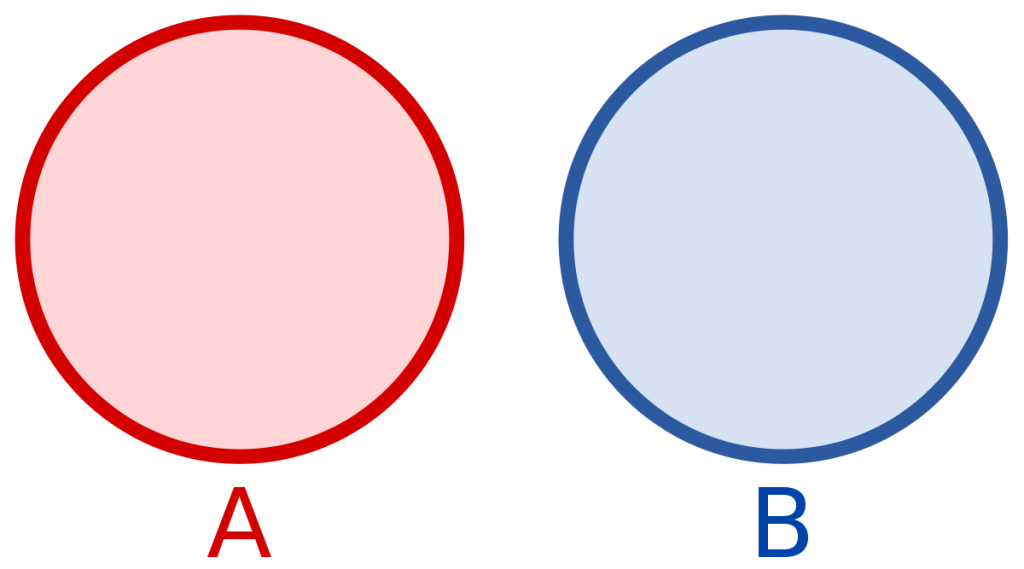Are Mutually Exclusive Events Independent?

# Are Mutually Exclusive Events Independent?

In probability theory, two events are said to be mutually exclusive or disjoint events if and only if they can not occur at the same time. For example, while tossing a coin head and tail can not occur at the same time. To understand the independence of events you can check my previous article. In short, Event A and B are independent if and only if P(A ∩ B) = P(A) x P(B). To be independent in mutually exclusive case either P(A) = 0 or P(B) = 0. If probability of both events is greater than Zero than it does not satisfy the condition for independence.

Don’t confuse independence with disjoint of events. Independence of events are completely different type of property.

Suppose P(A) > 0 and P(B) > 0 P(A)P(B) > 0. Then  P(A ∩ B) ≠ P(A) x P(B).For Example:

If event A and event B are mutually exclusive having P(A) = 1/2 and P(B) = 1/2. Find if event A and event B are independent?
Solution:

P(A) = 1/2

P(B) = 1/2

For independence Check:

P(A ∩ B) = P(A) x P(B) = 1/2 * 1/2 = 1/4 > 0.

So, Event A and Event B are not independent.

If A and B are mutually exclusive events, where each event has a non-zero probability of occurring then A and B are not independent.

NOTE: Don’t confuse independence with disjoint of events. Independence of events are completely different type of property.